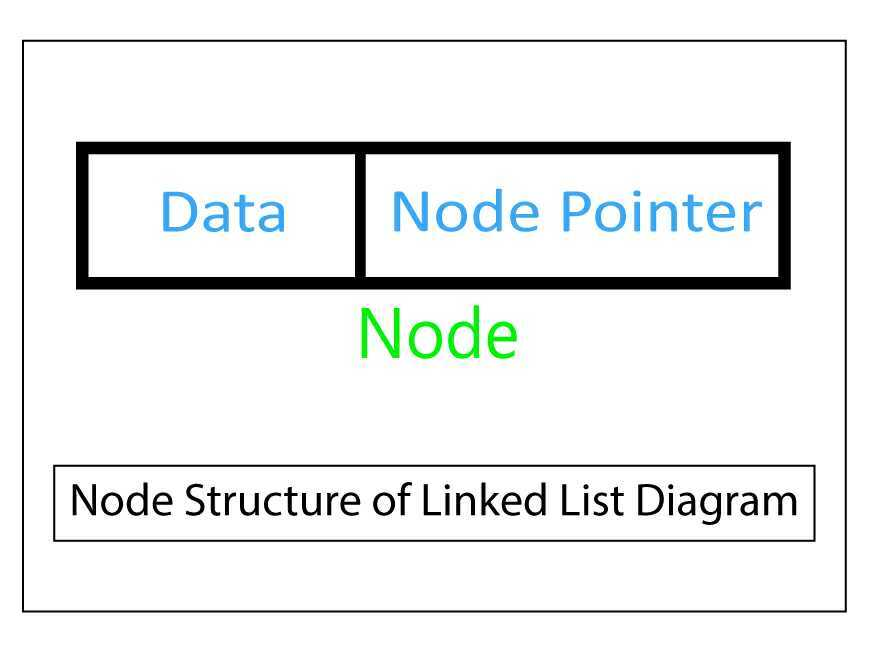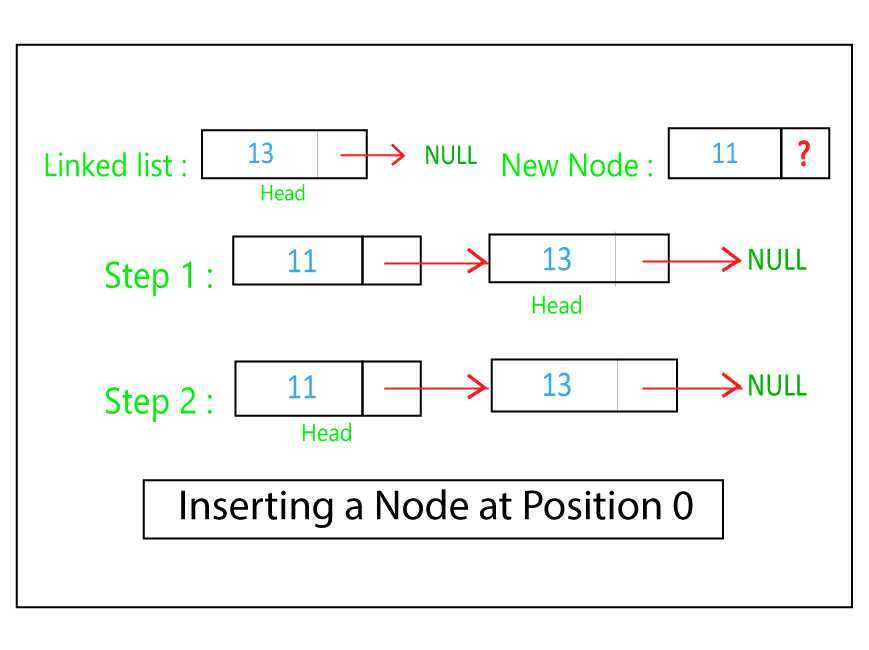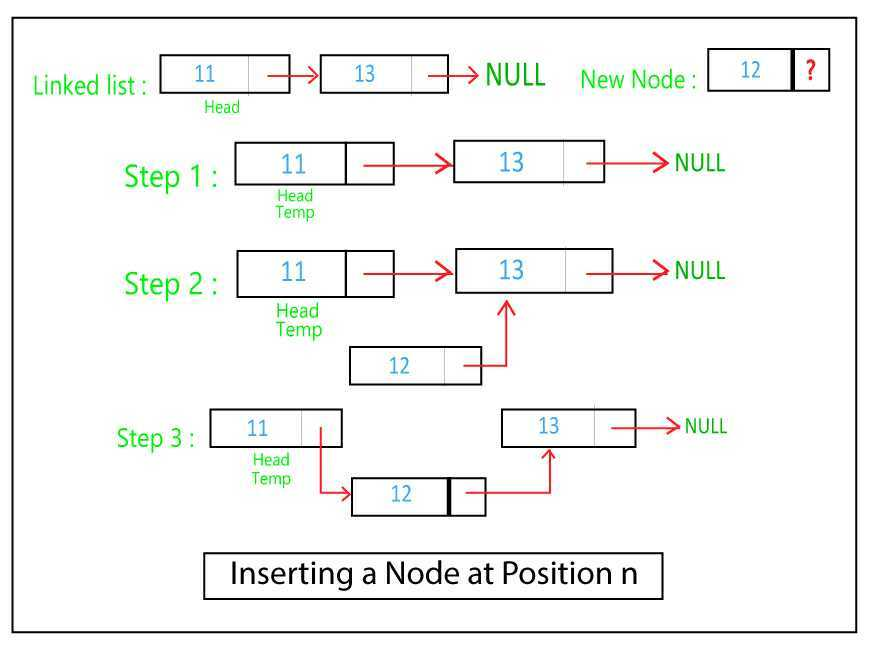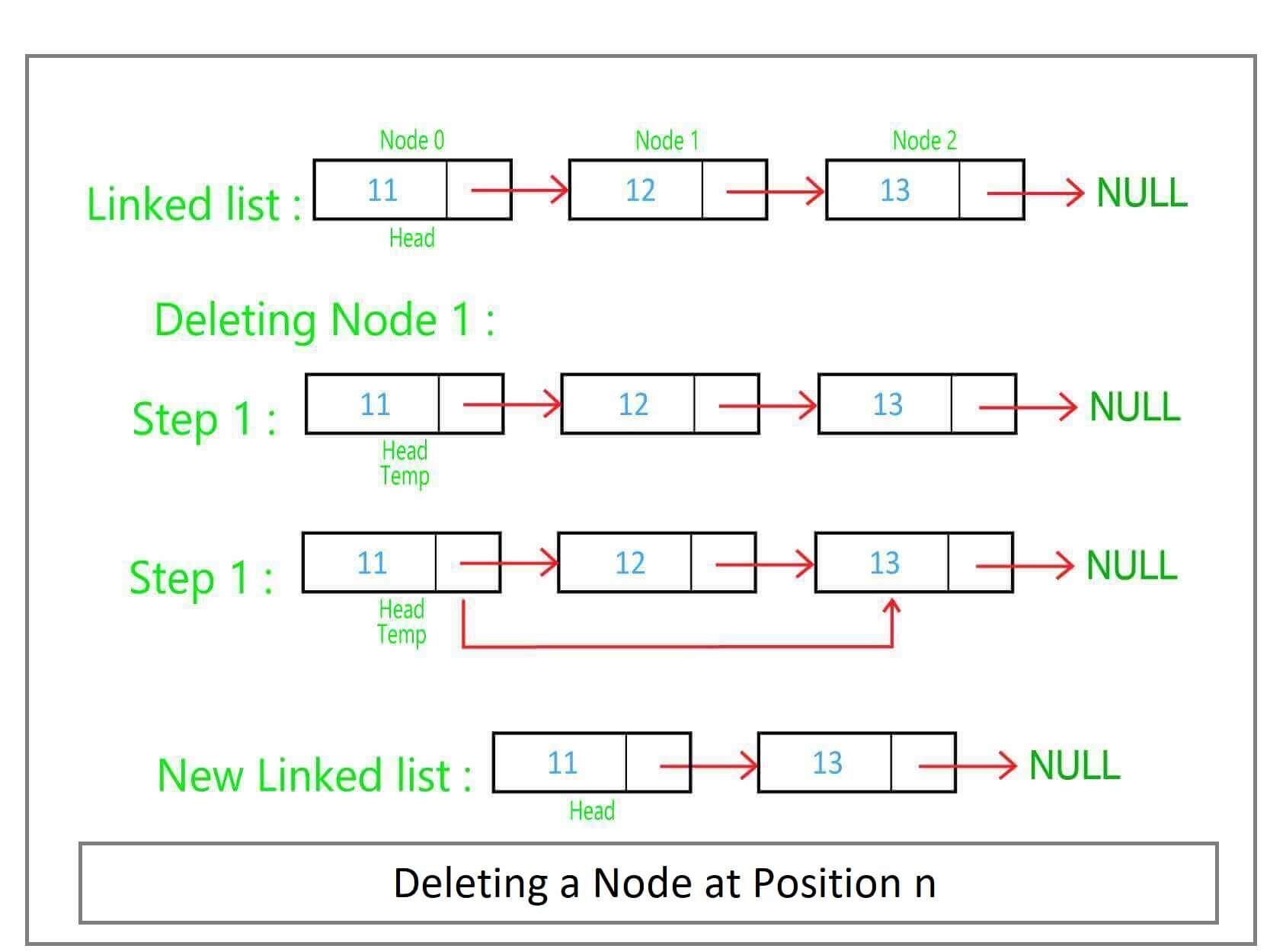A linked list is a data structure that implements the storage of data elements in a consecutive manner. Linked lists can be used to implement the queue data structure. There are three main types of linked lists – singly linked list, doubly linked list, and circular linked lists. A singly linked list consists of a chain of nodes, where each node has a data element and a pointer to the memory location of the next node. This is best demonstrated by the figure above. This post will implement the singly linked list data structure using C++.

#### Nodes in a Linked List

As mentioned, a linked list consists of discrete elements that are nodes. To use such a node in our linked list, a node structure is created. Structures are used to create user-defined data types in C++. A node structure contains a data element of an integer type and a pointer element to the next node structure. To create a linked list the first element points to the next element, the second to the third and so on, until the last element which points to NULL, indicating the end of the linked list.```struct NODE {
int data;
NODE *next;
};
```

Now that the structure of a node is ready to be used, a linked list data structure can be implemented using the concept of classes in C++. The most important element of the singly linked list is the first element in the list, commonly referred to as the head element. This is because the first element is the beginning of the link that helps find all the other nodes. It is not possible to find an intermediate node in a linked list without the head node.

```classLinkedList {
private:
public:
}
};
```

The above code declares a head pointer of the NODE structure as a private data member. The constructor instantiates the head pointer to NULL. This indicates that when an object of the LinkedList class is created, the first element, pointed to by head is a NULL element by default.

#### Insert Operation

To insert a node into a linked list two essential parameters are needed: the data element of the new node and its position in the linked list. First, a pointer to the new_nodeis created using the NODE structure and its data element is assigned the value as given by the user. Next, it is important to note that inserting a node at the beginning of a linked list i.e. at position 0, is unlike inserting a node at some other position.

If the node has to be inserted at the beginning of the linked list (position 0), the next element of the new_nodeshould point to the head of the linked list. The head element must also be updated as now the new­_nodeis the head of the linked list.However, a node can also be inserted at any other position, for example, position n. For this, a traversal of the linked list is required and a temp pointer is created which initially points to the head of the linked list. This pointer traverses n-1 nodes until it reaches the node whose next element should be the new_node. First, the next element of the new_node is made to point to temp’s next element, following which temp points to the new_node. This ensures that the previous element points to the new node and the new node points to the next element thus maintaining the chain in the linked list. This operation is demonstrated by the following figure.The insert_node()method given below implements the above logic. The if-else condition statements check whether the insert position is at the beginning or not.

```voidinsert_node(int position, int value) {
NODE *new_node=new NODE;
new_node->data = value;
if (position ==0) {
}
else {
while (position !=1) {
temp= temp->next;
position-=1;
}
new_node->next = temp->next;
temp->next =new_node;
}
}
```

#### Delete Operation

To delete a node from a linked list, its position in the linked list is the required parameter. Similar to the insert operation, deleting the first node is different from deleting a node at any other position in the linked list. To delete the first node from the linked list, the head element needs to be changed to the second element which can be accessed using the next pointer of the current head element. However, to delete a node from any other position in the linked list, it’s previous node must be updated. Again, to delete a node from position n, the temp pointer traverses over n-1 nodes of the linked list. The next element is then updated to point the next element of the next element i.e. skipping over the node expected to be deleted. This is demonstrated by the following figure.The delete_node()method given below implements the logic for deleting a node from a linked list.

```voiddelete_node(int position) {
if (position ==0) {
}
else {
while (position !=1) {
temp= temp->next;
position-=1;
}
temp->next = temp->next->next;
}
}
```

#### Display Operation

The display operation is the simplest operation of a linked list. A temp pointer is created that corresponds to the head of the linked list. Next, the data element of the pointer is printed while the pointer is made to point to the next element iteratively until the last element i.e. NULL is reached. This operation allows the traversal of the complete linked list from its head to the last NULL element. This is implemented by display() method given below.

```voiddisplay() {
while (temp !=NULL) {
cout<<temp->data<<"->";
temp= temp->next;
}
cout<<"NULL"<<endl;
}
```

#### Complete Code for Linked List C++

```// C++ Implementation of Singly Linked List
#include
usingnamespacestd;

struct NODE {
int data;
NODE *next;
};

private:
public:
}
voidinsert_node(int position, int value) {
NODE *new_node=new NODE;
new_node->data = value;
if (position ==0) {
}
else {
while (position !=1) {
temp= temp->next;
position-=1;
}
new_node->next = temp->next;
temp->next =new_node;
}
}
voiddelete_node(int position) {
if (position ==0) {
}
else {
while (position !=1) {
temp= temp->next;
position-=1;
}
temp->next = temp->next->next;
}
}
void display() {
while (temp !=NULL) {
cout<<temp->data<<"->";
temp= temp->next;
}
cout<<"NULL"<<endl;
}
};

intmain() {
list.display();
list.insert_node(0, 13);
list.display();
list.insert_node(0, 11);
list.display();
list.insert_node(1, 12);
list.display();
list.delete_node(0);
list.display();
list.delete_node(1);
list.display();
return0;
}
```

#### Output

```NULL
13->NULL
11->13->NULL
11->12->13->NULL
12->13->NULL
12->NULL
```

Implementing data structures such as linked list are essential concepts in programming. If you find yourself stuck on a particular bug or would like to receive further clarification on this topic,FavTutor is always here to provide you with help from expert tutors 24/7. Get started by sending a message through the chat on the bottom right. Happy programming!